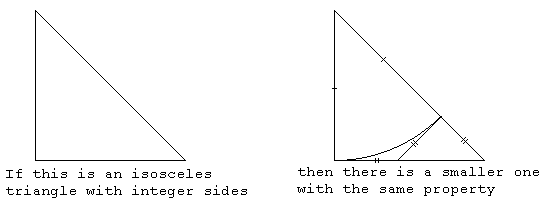The square root of 2 can never be written exactly as a fraction. In other words,

How can we show this? Here are four proofs.

## Algebra

The usual way is as follows.

• Suppose $\sqrt{2}$ can be written as a fraction:
• $\sqrt{2}=a/b$
• Then $2=a^2/b^2$
• Then $2b^2=a^2$
• Then $a^2$ is even
• Hence $a$ is even
• (This takes a small proof of its own.)
• So we can write a=2k
• Hence $2b^2=(2k)^2=4k^2$
• So $b^2=2k^2$
• So now $b^2$ is even
• Hence $b$ is even
• So now we can reduce $\frac{a}{b}$ to a smaller fraction.
• Lather, rinse, repeat, as they say.
• Following this process we can get smaller and smaller fractions, each of which is exactly the square root of two. Clearly this is nonsense.
• The argument is sound, so the premise must be false.
• Thus we can never write $\sqrt{2}$ as $\frac{a}{b}$

## Geometry

Suppose we can draw a square that has integral sides and an integral diagonal. Using Pythagoras' theorem we can see that this is equivalent to saying that $\sqrt{2}$ is rational.

Consider the following diagram:By Pythagoras we know that if the sides are $a$ and the diagonal is $b$ then $2a^2=b^2.$ This diagram shows that given such a pair, $a,b,$ we can find a smaller pair. (Exercise for the reader: show that $a$ and $b$ in the smaller pair are still integers.)

Repeat.

Eventually we run out of numbers and thus complete our proof by contradiction

## Algebra, again

 Exercises for the diligent reader: Show that $(2a-b)^2=2(b-a)^2$ Show that b-a < a what's the relationship between this proof and the previous geometrical one?
Suppose $b^2=2a^2$ where $a,b$ are integers and as small as possible. Then a little algebra shows that $(2a-b)^2=2(b-a)^2$ so that $(b-a,2a-b)$ is a new pair of integers with the same property. But $b-a\lt~a$ and so we've got a smaller pair of integers whose ratio is $\sqrt{2},$ contrary to our original decision to take the smallest pair.

## Continued Fractions

 Another exercise for the diligent reader: Show that for every number of the form $a/b,$ its continued fraction will terminate.
Every rational number has a finite continued fraction. We show now that the continued fraction for $1+\sqrt{2}$ does not terminate. That means $1+\sqrt{2}$ is irrational, and hence $\sqrt{2}$ is irrational.

To compute the continued fraction we separate the target number into its integer part and the fractional part

• $T=1+\sqrt{2}=2+\epsilon$ where $\epsilon=\sqrt{2}-1$
$\epsilon$ is between 0 and 1 as required, so we can take its reciprocal, giving $\frac{1}{\sqrt{2}-1}$ which after rationalising the denominator is $1+\sqrt{2}.$ That's what we started with, so the continued fraction for $1+\sqrt{2}$ is:

• $\LARGE{1+\sqrt{2}=2+\frac{1}{2+\frac{1}{2+\frac{1}{2+\frac{1}{2+...}}}}}$
Thus the continued fraction for $1+\sqrt{2}$ is [2;2,2,2,2,...] which does not terminate. Hence $1+\sqrt{2}$ is irrational, and so $\sqrt{2}$ is irrational.

CategoryMaths
AbsoluteValue
AlgebraicNumber
ApproximatingPi
CauchySequence
ContinuumHypothesis
CountableSet
DedekindCut
Denominator
DiophantineEquation
DividingRationalNumbers
DividingWholeNumbers
Divisor
DomainOfAFunction
EuclideanAlgorithm
FermatsLastTheorem
ImproperFraction
IrrationalNumber
MagnitudeOfAVector
MathematicsTaxonomy
MixedNumber
MultiplyingRationalNumbers
Numerator
PiIsIrrational
PythagorasTheorem
RealNumber
ReducingFractionsToLowestTerms
SubtractingRationalNumbers
Thales
TypesOfNumber
(none) (none)
ContinuedFraction
Pythagoras
RationalNumber
(none)

## You are here

RootTwoIsIrrational
Denominator
Integer
PythagorasTheorem
SquareRoot
WholeNumber
CauchySequence
CountableSet
FamousPeople
IrrationalNumber
ReducingFractionsToLowestTerms
Thales
Numerator
RealNumber
CoDomainOfAFunction
ComplexConjugate
ComplexNumber
ComplexPlane
CountingNumber
DifferenceOfTwoSquares
DomainOfAFunction
Euler
Function
ImageOfAFunction
ImaginaryNumber
NaturalNumber
PolarRepresentationOfAComplexNumber
RiemannSurface
Root